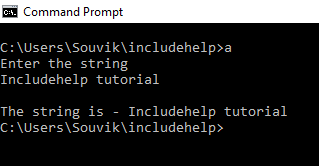# getchar() function in C language with Example

Here, we are going to learn about the getchar() function of library function stdio.h in C language with its syntax, example.
Submitted by Souvik Saha, on February 21, 2019

## getchar() function in C

The getchar() function is defined in the <stdio.h> header file.

Prototype:

```    int getchar(void);
```

Parameters: FILE *filename(for file handling), otherwise it will be void.

Return type: int

Use of function:

In the file handling, through the getchar() function we take the character from the input stream stdin. The prototype of the function getchar() is int getchar(void);

The character which is read is an unsigned char which is converted to an integer value. In the case of file handling, it returns EOF when end-of-file is encountered. If there is an error then it also returns EOF.

## getchar() example in C

```#include <stdio.h>
#include <stdlib.h>

int main()
{
//Initialize the character array
char str;
int i = 0, j = 0;

printf("Enter the string into the file\n");
//takes all the characters until enter is pressed
while ((str[i] = getchar()) != '\n') {
//increment the index of the character array
i++;
}

//after taking all the character add null pointer
//at the end of the string
str[i] = '\0';
printf("\nThe file content is - ");
//loop is break when null pointer is encountered
while (str[j] != '\0') {
//print the characters
putchar(str[j]);
j++;
}

return 0;
}
```

Output